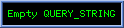Fabrizio Iacopetti's Web site
 Best view with 1024 x 768 Home Mail-me

 A few notes concerning C++ integral data types
 You are the visitor n.ContentsOn this page, a few sides of dealing with whole numbers in C++ are described. In particular, a few notes and examples are reported that could help avoiding mistakes while using integers.

 Increment/decrement, addition/subtractionSuppose you want to perform a certain operation 40000 times; suppose you write the following C++ program. If you are aware that you need a four byte integer, but you don't check if the int type is really stored in 4 bytes, and your compiler stores int numbers in two bytes, then the programint a=0;for (int a=0; a<40000; a++){// operations to be done}results in an infinite loop, as after 32767 comes ... -32768, -32767 ...It is possible that the compiler warns you, if in the program the constant value 40000 is assigned to a variable that can't contain it.In this case you must take care to use an integer type stored at least on 4 bytes, for example you could try with the long long int type. Remember this was is example; as another example, in DevCpp 4.9.8.0 for Win an int is on 4 bytes and a long long int is on 8 bytes.Take care: in general, the long int type is stored either in as many bytes as the int type, or in more bytes: check your implementation.Even if you have taken care that your variables fit into the type you have choosen for them, their sum or subraction could generate a result out of bounds allowed for that type, and you cannot recognize it at run-time (unless you try managing overflow, carry and so on). Maybe it could be useful to use "larger" types to store the result of such operations.Similar thing (much more frequent) when you multiply two int; if you are sure that every possible result can fit into an int, you can use an int to store the product, otherwise you should use a "larger" type. With a 4 byte int type, for which bounds are -2147483648 and +2147483647, let's write the following program:int a= 200000; int b=200000;cout << a*b << endl;int c=2000000000;if (a*b < c){cout << "success\n";}else{cout << "failure\n";}What do you think is the result? The program shows on your screen "success", and a*b is showed to be 1345294336 by the cout operator, instead of 40000000000.You should have used a long long int for c and assign a*b to a long long int d, provided the long long int is on eight bytes.

 Absolute valueWhen you use the int abs(int n) function, due to the dissymetrical representation interval of integers in two's complement, you should take care that n is not the minimum allowed integer; in fact, supposing a short int is on two bytes, the following program does not work.short int asi = 0;asi = -32768;short int aaa=abs(asi);cout << "asi = " << asi << " , aaa = abs(asi) = " << aaa << endl;As abs(-32768) cannot be represented on two bytes, the abs function returns -32768, so you get on the screenasi = -32768 , aaa = abs(asi) = -32768If you wish you can contact me for informations and suggestions at: